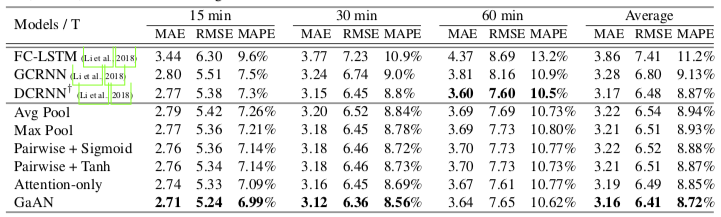# GaAN: 用于大型图与时空图的门控注意力网络

## 三种类型 Graph Aggregator 的对比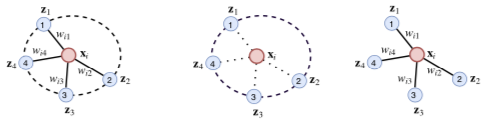• 实线边表示中心节点对邻居节点汇聚来的信息加以不同权重
• 虚线圆表示邻居节点相互之间会影响汇聚出的信息的权重
• Attention Aggregator 是后两者的结合：边对应打分，圆对应 Softmax

## Attention Aggregator 的例子

• x -> q
• $N_x$ -> K, V （其中 V 的生成用了 LeakyReLU）
• 加权和：$\text{H} = V \text{softmax}(K^T q)$
• 多头组合：$y(x) = F([x, h_1, \cdots, h_k])$（F 代表一个全连接层）

• GAT 使用普通的注意力而非键值注意力
• GAT 的打分函数是拼接后用全连接层而非点积模型

## Gated Attention Network

GaAN 相对一般 Attention Aggregator 的改进在于给各头加权（Gated）：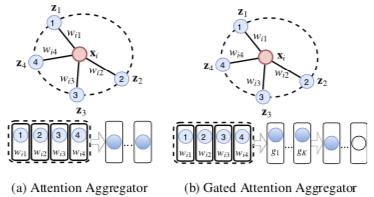• 加权多头：$y(x) = F([x, g_1 h_1, \cdots, g_h h_k])$
• $g(x) = \text{sigmoid}(F_1([x, \max(\{F_2(z)| z \in N_x\}), \text{mean}(N_x)]))$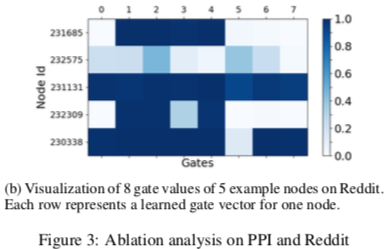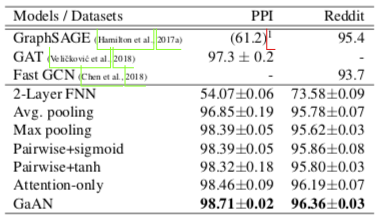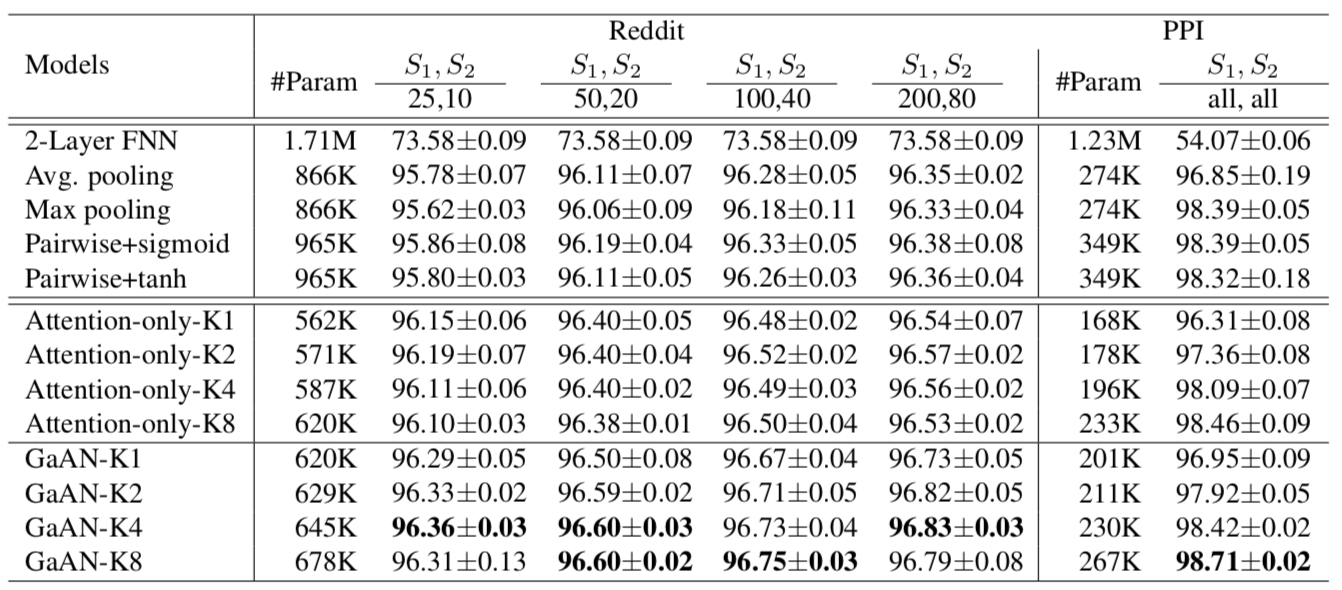## Graph GRU

• 整体是个 GRU 模型，内部计算使用图的 Graph Aggregator（可以不是 GaAN）
• 更新门 U 和重置门 R 都是 (X 聚合 X) + (X 聚合 H) 再激活的结果，其中 X 是该时间各节点的输入，H 是该时间各节点的隐状态

1. FC-LSTM：LSTM 里套 FC
2. GCRNN：LSTM 里套 ChebNet（GCN 的 Spectal-based 原型）
3. DCRNN：GRU 里套 DGC（把边归一化为转移概率的 GCN）
4. Graph GRU：GRU 里套本文总结的那些 Graph Aggregator